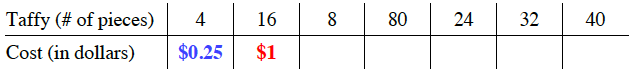### Home > CCAA > Chapter 8 Unit 7 > Lesson CC2: 8.1.1 > Problem8-12

8-12.

Four pieces of taffy cost $25$ cents. Complete the table below.  Homework Help ✎

 Taffy (# of pieces) $4$ $16$ $8$ $80$ $24$ $32$ $40$ Cost (in dollars)

What can you multiply $4$ by to get $16$ pieces of taffy?

$0.25(4)=1$ dollar for $16$ pieces of taffyNow use this same method to fill in the rest of the table.